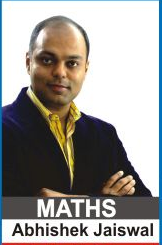Maths Lectures by Abhishek Jaiswal (B.Tech IIT Roorkee) for class 12th Students. The lectures cover theory both for CBSE and Competetive Examinations.

For Queries and Live online batches contact 9312922770.Lecture 1 | Probability | Introduction

Lecture 2 | Probability | Conditional Probability

Lecture 3 | Probability | Total Probability

Lecture 4 | Probability | Problem Solving

Lecture 5 | Probability | Bayes' Theorem

Lecture 6 | Probability | Bernoulli's Trials

Lecture 7 | Probability

Lecture 8 | Probability

Lecture 9 | Probability | Geometric Probability